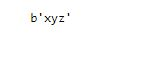/  Technology   /  Python Convert String to bytes## Python Convert String to bytes

This article will guide you on how to convert string to bytes in python.

#### Example 1:

String ‘abc’ is converted to bytes using below example.

```s = 'abc'
print(type(s))

# string to bytes using bytes()
b = bytes(s, encoding='utf-8')

print('After encoding to bytes =', b)
print(type(b))```

Output:#### Example 2:

Consider string ‘xyz’ and we are converting this string to bytes.You can also encode in the below manner.

`bytes('xyz', encoding='utf-8')`

Output: